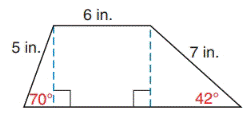Chapter 11.4, Problem 16E### Elementary Geometry for College St...

6th Edition
Daniel C. Alexander + 1 other
ISBN: 9781285195698

#### Solutions

Chapter
Section### Elementary Geometry for College St...

6th Edition
Daniel C. Alexander + 1 other
ISBN: 9781285195698
Textbook Problem
5 views

# In Exercises 15 and 16, find the area of the given figure. Give the answer to the nearest tenth of a square unit.Trapezoid

To determine

To find:

The area of the given figure

Explanation

Approach:

Finding the area of the figure is followed by identifying the figure and find area with the suitable geometry formulae.

Calculation:

Given,

The area of the given figure (A)= The area of the triangle (MRQ)(A1)+ The area of the square RSPQ(A2)+ The area of the triangle SNP(A3)

The area of the triangle (MRQ)(A1):

For ΔRMQ

α+β+γ=180

Here α=70 and γ=90

70+β+90=180

160+β=180

160+β160=180160

β=20

Here,

β=20

a=5 in.,

c=6 in.

Area of the triangle (A1)=12acsinβ

12(5)(6)sin20

=(5)(3)sin20

=(15)sin20

=(15)(0.34)

A1=5.1 in2.

The area of the square (RSPQ)(A2):

Side (s)=6 in.

### Still sussing out bartleby?

Check out a sample textbook solution.

See a sample solution

#### The Solution to Your Study Problems

Bartleby provides explanations to thousands of textbook problems written by our experts, many with advanced degrees!

Get Started

#### Find more solutions based on key concepts